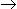# Chemical Engineering - Chemical Reaction Engineering

Exercise : Chemical Reaction Engineering - Section 3
1.
Space velocity
describes the extensive operating characteristics of a tubular flow reactor.
is the maximum feed rate pre unit volume of reactor for a given conversion.
is a measure of the ease of the reaction.
all (a), (b) and (c)
Explanation:
No answer description is available. Let's discuss.

2.
The rate of the chemical reaction AB doubles as the concentration of A i.e.., CA is doubled. If rate of reaction is proportional to CAn, then what is the value of n for this reaction ?
0.5
1
0
2
Explanation:
No answer description is available. Let's discuss.

3.
A reaction which is catalysed by a base is catalysed by all substances which have a tendency to
lose a proton
gain a proton
gain an electron
none of these
Explanation:
No answer description is available. Let's discuss.

4.
For a homogeneous reaction of nth order, the dimension of the rate constant is given by
l/(time)n
(concentration)1 - n/(time)
(concentration)n - 1/(time)
none of these
The fractional volume change between no conversion and complete conversion, for the isothermal gas phase reaction, 2AR, is